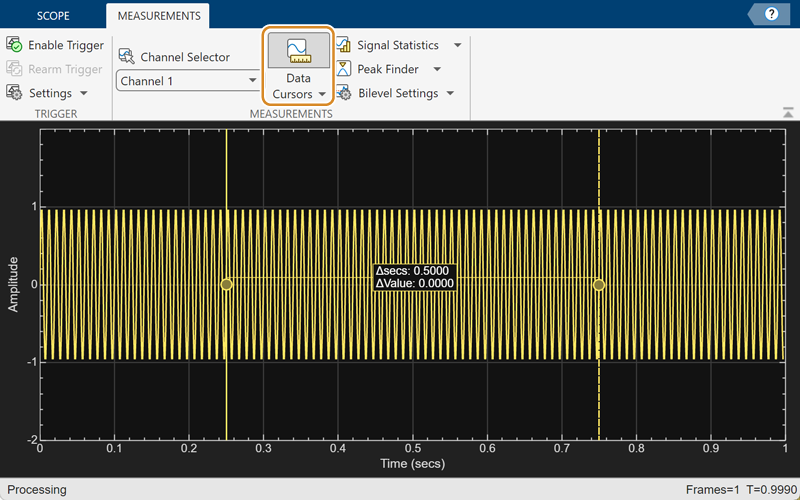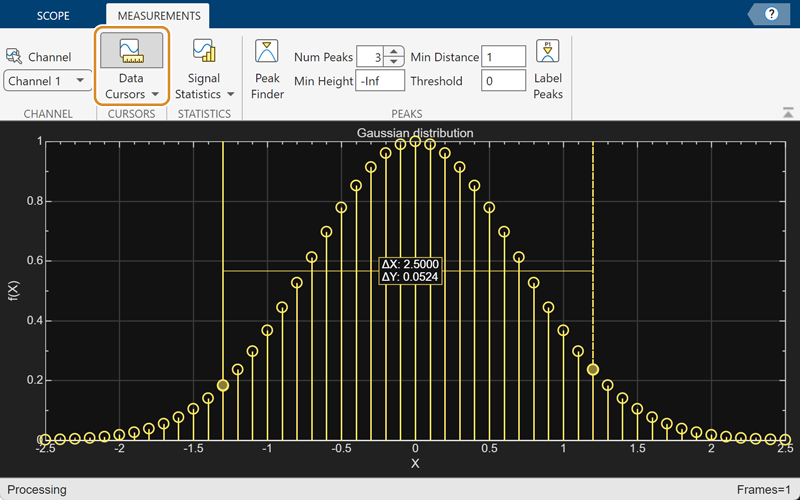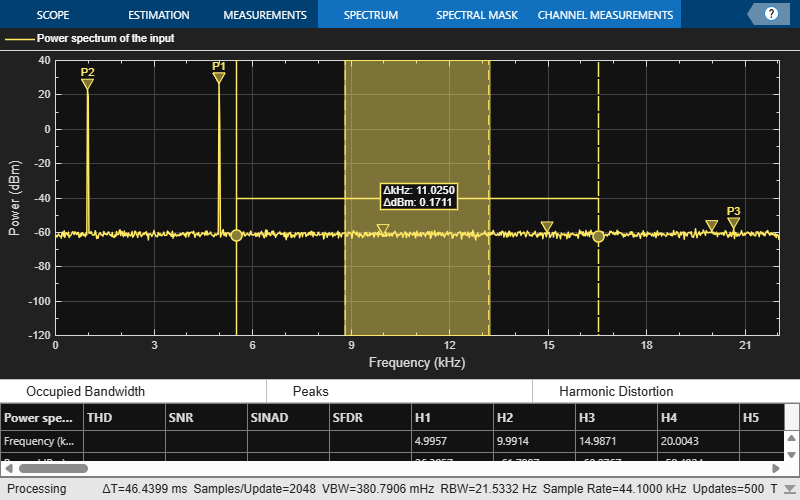# CursorMeasurementsConfiguration

Display screen or waveform cursors

## Description

Use the `CursorMeasurementsConfiguration` object to enable screen or waveform cursors. You can control the cursor settings from the toolstrip of the scope or from the command line.

To modify the cursor settings in the scope UI, click the Measurements tab and enable Data Cursors. Each cursor tracks a vertical line along the signal. The scope displays the difference between x- and y-values of the signal at the two cursors in the box between the cursors.

Spectrum Analyzer ToolstripTime Scope ToolstripArray Plot Toolstrip## Creation

### Syntax

``cursormeas = CursorMeasurementsConfiguration()``

### Description

example

````cursormeas = CursorMeasurementsConfiguration()` creates a cursor measurements configuration object. ```

## Properties

expand all

All properties are tunable.

x-coordinates of the cursors, specified as a two-element vector of real elements.

Data Types: `double`

Snap cursors to data, specified as `true` or `false`.

#### Scope Window Use

Click the Measurements tab on the scope toolstrip. In the Cursors section, click Data Cursors, and then select the Snap to data parameter.

Data Types: `logical`

Enable cursor measurements, specified as `true` or `false`. Set this property to `true` to enable cursor measurements.

#### Scope Window Use

Click the Measurements tab on the scope toolstrip. In the Cursors section, select Data Cursors.

Data Types: `logical`

## Examples

collapse all

Create a sine wave and view it in the Time Scope. Enable data cursors programmatically.

Initialization

Create the input sine wave using the `sin` function. Create a `timescope` MATLAB® object to display the signal. Set the `TimeSpan` property to 1 second.

```f = 100; fs = 1000; swv = sin(2.*pi.*f.*(0:1/fs:1-1/fs)).'; scope = timescope(SampleRate=fs,... TimeSpanSource="property",... TimeSpan=1);```

Data Cursors

Enable data cursors in the scope programmatically by setting the `Enabled` property of the `CursorMeasurementsConfiguration` object to `true`.

```scope.CursorMeasurements.Enabled = true; scope(swv); release(scope)```Compute and display the power spectrum of a noisy sinusoidal input signal using the `spectrumAnalyzer` MATLAB® object. Measure the peaks, cursor placements, adjacent channel power ratio, and distortion values in the spectrum by enabling these properties:

• `PeakFinder`

• `CursorMeasurements`

• `ChannelMeasurements`

• `DistortionMeasurements`

Initialization

The input sine wave has two frequencies: 1000 Hz and 5000 Hz. Create two `dsp.SineWave` System objects to generate these two frequencies. Create a `spectrumAnalyzer` object to compute and display the power spectrum.

```Fs = 44100; Sineobject1 = dsp.SineWave(SamplesPerFrame=1024,PhaseOffset=10,... SampleRate=Fs,Frequency=1000); Sineobject2 = dsp.SineWave(SamplesPerFrame=1024,... SampleRate=Fs,Frequency=5000); SA = spectrumAnalyzer(SampleRate=Fs,SpectrumType="power",... PlotAsTwoSidedSpectrum=false,ChannelNames={'Power spectrum of the input'},... YLimits=[-120 40],ShowLegend=true); ```

Enable Measurements Data

To obtain the measurements, set the `Enabled` property to `true`.

```SA.CursorMeasurements.Enabled = true; SA.ChannelMeasurements.Enabled = true; SA.PeakFinder.Enabled = true; SA.DistortionMeasurements.Enabled = true;```

Use `getMeasurementsData`

Stream in the noisy sine wave input signal and estimate the power spectrum of the signal using the spectrum analyzer. Measure the characteristics of the spectrum. Use the `getMeasurementsData` function to obtain these measurements programmatically. The `isNewDataReady` function returns `true` when there is new spectrum data. Store the measured data in the variable `data`.

```data = []; for Iter = 1:1000 Sinewave1 = Sineobject1(); Sinewave2 = Sineobject2(); Input = Sinewave1 + Sinewave2; NoisyInput = Input + 0.001*randn(1024,1); SA(NoisyInput); if SA.isNewDataReady data = [data;getMeasurementsData(SA)]; end end```The right side of the spectrum analyzer shows the measurement panes you enabled. The values in these panes match the values in the last time step of the `data` variable. You can access the individual fields of `data` to obtain the various measurements programmatically.

Compare Peak Values

Use the `PeakFinder` property to obtain peak values. Verify that the peak values in the last time step of `data` match the values shown on the spectrum analyzer plot.

`peakvalues = data.PeakFinder(end).Value `
```peakvalues = 3×1 26.9261 24.1149 -46.3163 ```
`frequencieskHz = data.PeakFinder(end).Frequency/1000`
```frequencieskHz = 3×1 4.9957 0.9905 0.0646 ```

## Version History

Introduced in R2022a